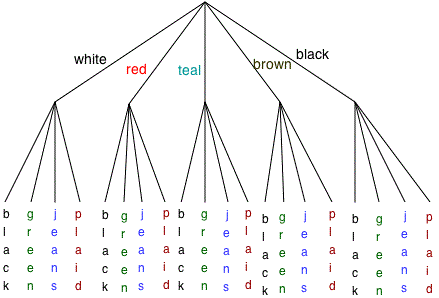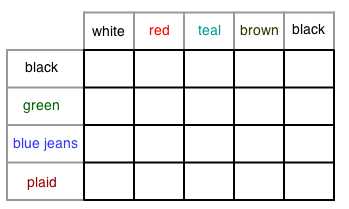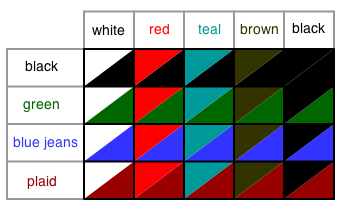### Home > GC > Chapter 4 > Lesson 4.2.4 > Problem4-82

4-82.

Choose a method to represent the following problem and use it to answer the questions below.

Kiyomi has $4$ pairs of pants (black, green, blue jeans, and plaid) and she has $5$ shirts (white, red, teal, black, and brown).

1. If any shirt can be worn with any pair of pants, how many outfits does she own? That is, how many different combinations of pants and shirts can she wear?

2. What is the probability that she is wearing black?

We have several different modes of representation we can use.Each branch represents the same probability as one cell in the area model.

($5$ shirts)($4$ pairs of pants) = $20$ outfits

Each column represents $\frac{1}{5}$ probability, each row represents $\frac{1}{4}$ probability, and each cell represents $\frac{1}{20}$ probability.

The probability of Kiyomi wearing black can be calculated by counting the number of cells containing black (the number of outfits with black) and multiplying by the probability of each outfit.